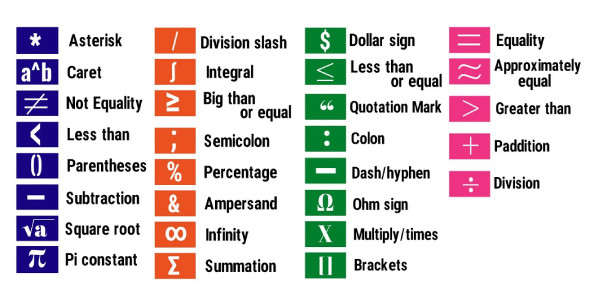# Say IT WITh Symbols Test: Quiz!

16 Questions | Total Attempts: 159SettingsAre you someone who gets all excited when they see a math problem just waiting to be solved? In the trivia quiz below dubbed say it with symbols, you will be expected to answer the questions to the best of your ability. Feel like you are up for the challenge? What are you waiting for? Press start, and let's have some fun!

• 1.
3x + 1 + 8x + 9
• A.

12x + 10

• B.

5x + 8

• C.

11x + 11

• D.

11x + 10

• 2.
2x + 5y - 7x + 8y
• A.

9x + 13y

• B.

5x + 3y

• C.

-5x + 3y

• D.

-5x + 13y

• 3.
4(x + 8) - 9
• A.

4x + 32

• B.

4x + 23

• C.

12x - 9

• D.

4x + 24

• 4.
-3(x + y) + 5(x - y)
• A.

2x - 8y

• B.

-2x + 2y

• C.

8x - 8y

• D.

2x - 8y

• 5.
2x + 5y - 7x + 8y
• A.

9x + 13y

• B.

5x + 3y

• C.

-5x + 3y

• D.

-5x + 13y

• 6.
4(x + 8) - 9
• A.

4x + 32

• B.

4x + 23

• C.

12x - 9

• D.

4x + 24

• 7.
-3(x + y) + 5(x - y)
• A.

2x - 8y

• B.

-2x + 2y

• C.

8x - 8y

• D.

2x - 8y

• 8.
Which expressions are Linear?
• A.
• B.
• C.
• D.
• E.
• 9.
• A.
• B.
• C.
• D.

1

• 10.
• A.
• B.
• C.
• D.

1

• 11.
Which expression(s) represent the area of the model above?
• A.

X(x+11)

• B.

X(11x)

• C.
• D.

2x + 11

• 12.
Which of the expression(s) represent the area model above?
• A.
• B.
• C.
• 13.
The Metropolis Middle School volleyball team operates the concession stand at school basketball games to help raise money for new uniforms.  I = 10x + 2 E = 3x + 5 Which equation(s) show Profit?
• A.

P= I - E

• B.

P= (10x + 2) - (3x + 5)

• C.

P= 7x - 3

• D.

P= 10 + 2 - 3x + 5

• 14.
The Metropolis Middle School volleyball team operates the concession stand at school basketball games to help raise money for new uniforms.  I = 10x + 2 E = 3x + 5 Using the equation P = (10x + 2) - (3x + 5) , Find out the profit if the volleyball team sold 13 items:
• A.

88

• B.

98

• C.

52

• 15.
The Metropolis Middle School volleyball team operates the concession stand at school basketball games to help raise money for new uniforms.  I = 10x + 2 E = 3x + 5 Using the equation P = (10x + 2) - (3x + 5) , How many items were sold if the Profit was \$60:
• A.

7.6

• B.

9

• C.

8

• D.

10

• 16.
3x + 1 + 8x + 9
• A.

12x + 10

• B.

5x + 8

• C.

11x + 11

• D.

11x + 10

Related TopicsBack to top